##### Typology of Foundations

In general, foundations of typical tensile buildings can be classified depending on the load to be resisted, in any of these types:

1. Vertical pulling load (vertical cable)
2. Leaning pulling load (any cable or guy-rope fixed to the ground)
3. Leaning pushing load (tilted masts, base of an arch, etc.).
4. Vertical pushing load (vertical mast)
5. Bending moment, with vertical and horizontal loads of lesser importance (especially vertical columns supporting a membrane, without stays or guy-ropes)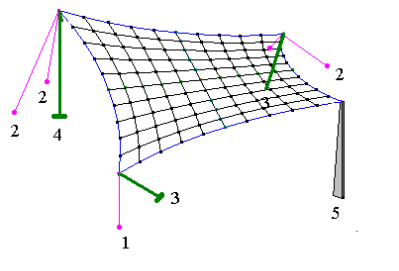As we can see, only type 4 is what we would call traditional foundation. Type 3 could be considered traditional if the horizontal component was very small or if it was possible to establish a grid of straps between these and other footings. If not, we’ll see that the design of the foundation is quite different.

##### Balance resources

To balance the loads produced by the structural elements of the tensile constructions, foundations use a different set of resources that, although they could be used in any type of foundation, are more common in this typology.

So, we can consider the following resources:

• The compressive strength of the soil at the bottom of the foundation.
• The compressive strength of the soil, at the sides of the foundation.
• The friction between the sides and the bottom of the foundation with the soil.
• The weight of the foundation and all other materials or structural elements located above the foundation: soil, base, slab, pavement, …
• The mechanical resistance of a possible continuous reinforced concrete slab, on the foundation.
##### Vertical compression resistance of the soil

Vertical compression loads are usually balanced only with the reaction of the soil at the base of the foundation. It is necessary that the distributed load on the surface of the foundation does not exceed a value σt which we call soil bearing capacity

Fv / A ≤ σt

This value σt, is obtained from the geotechnical study data of the soil and, obviously, it is obtained using the characteristic values of soil: soil density, angle of internal friction and cohesion. With this information and according to the size of the footing we can calculate the pressure that will be applied to the ground.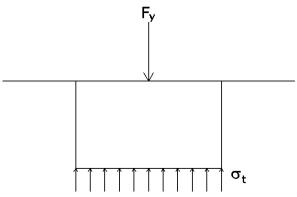Many times this value includes the safety factor, so that the dimensioning of the foundation is immediate.

So, if we are going to analyse a vertical column supporting a compressive load of 50 t, on a soil that has a bearing capacity of 1.5 kp / cm ² = 15 t / m², we will need a footing with an surface of 50 / 15 = 3.33 m² which corresponds approximately to a square footing of 1.85 m or 1.9 m per side. The height of the footing depends on other factors (if the footing must be stiff or flexible), but right now this is not relevant.

##### Horizontal compression resistance of the soil

When analyzing foundations of tensile constructions we often find horizontal loads that must be balanced. And most of the times we can count on only the soil resistance to balance these loads, so we need to determine which is the horizontal compression resistance of the soil.

It is not the bearing capacity of the soil, as it was in the previous case. Now soil resistance is given by the capacity of the mass of ground surrounding the foundation to resist horizontal displacements.

To determine the value of this capacity will have to turn to the general knowledge of Soil Mechanics. We know that soils are granular materials, and as with liquids, they cause a lateral pressure which depends on the load they support. Therefore, the denser a soil, the greater the lateral pressure it causes.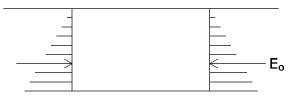If we dig a hole and fill it with a footing (usually concrete), this foundation is subjected to a lateral pressure, from all sides, produced by the surrounding soil. This pressure, called lateral earth pressure, varies with depth, because the deeper (more weight of soil on top) the greater the soil pressure.

This pressure on the lateral side of the foundation generates a force called earth pressure at rest E0. Not to be confused with the active earth pressure Ea. This is commonly used in the analysis of the retaining walls. It’s called this way since active earth pressure is generated at the precise moment that the wall begins to move, in the same sense of pressure. In the case of a foundation there is no displacement and therefore it makes no sense to speak about active earth pressure (Ka).

According to the Soil Mechanics theory, pressure at rest is developed as a force linearly distributed in triangular shape, with zero at the top, on the ground, and e0 at a depth h,

e0 = γ · h · K0

where γ is the soil density.

Thus the resultant load at rest, at a distance 2h/3 of the ground surface, is

E0 = ½ · K0 · γ . h²

where K0 is a value that directly depends on the angle of internal friction of soil Φ. It is generally accepted the following value

K0 = 1 – sin (Φ)

or other approximate values (K0 = 0.95 – sin (Φ)). This value is also affected by the degree of soil compaction.

K0 = 1 – sin (Φ) · √ Roc

being Roc the over consolidation ratio. We can take as normal the following values1:

• soft sands K= 0,50 to 0,65
• dense sands K= 0,35 to 0,45
• clay K= 0,70 to 0,75
• compacted sand K= 1,00 to 2,00
• compacted clay K= 0,80 to 1,50

Clearly, these values are highly variable; therefore the results we obtain in our calculations depend on its accuracy. It makes no sense to deal with very complex formulas, if we don’t know the value of K0 with a high degree of accuracy.

Understanding that this pressure is caused by the soil with no need of any movement, you can count on it safely. Not so with Eppassive earth pressure or passive resistancePassive resistance is the force needed to mobilize or move a soil (in our case, the one that surrounds the foundation) in the direction of the pressure itself. In this case, this force is well above the earth pressure at rest that we discussed earlier.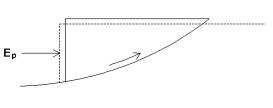However, we should not trust this passive resistance to balance external horizontal forces since it would mean to allow a not negligible movement of earth. This is not acceptable for normal construction, not either for tensile constructions although resultant problems might not be so severe.

The next figure (Figure 6.5 of Spanish Technical Code) shows a pattern of relationship between the pressure of the soil and the movements necessary for their development. It is important to emphasize the magnitude of these necessary movements to fully develop the passive pressure.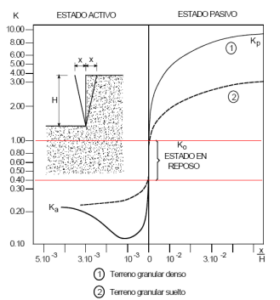However it is not a crazy idea to use this passive resistance as a resource to balance horizontal loads to accomplish the safety factor. If the earth pressure at rest is equal to the horizontal load, a safety factor fs = 1.5 means that passive resistance should be equal to 1.5 times the earth pressure at rest, what occurs practically in all soils. Moreover to mobilize a resistance equal to 1.5 times the earth pressure at rest, the displacement of soil is very small (a few millimetres) which is affordable for most tensile constructions.

##### The friction between the foundation and the soil

When two surfaces (in this case the footing and the ground bellow) are in contact and there is a force perpendicular (normal) to the plane of contact, friction generates a force that tries to prevent the displacement of one surface over the other.

The value of this frictional resistance Rf is equal to the normal force FN times the friction coefficient cf

Rf = FN · cf

In our case, the coefficient of friction between the ground and the foundation may not exceed the coefficient of internal friction of the soil. This value is the tangent of the angle of internal friction of the soil. So, it is essential to know this angle to evaluate the earth pressure at rest.

cf ≤ tg (Φ)

It is common practice to use a value of cf = 2/3 · tg (Φ). Nevertheless, there are cases where this value is not advisable, as it will be discussed below.

The frictional resistance between the soil and the footing is produced in two different situations, with different considerations:

1. Friction at the base of the foundation.

If at the base of the foundation there is a compressive load, it will cause a frictional resistance that will prevent lateral displacement of the foundation. For this to happen it is necessary that:

QT = QV + PF + PP > 0

where

QV    Vertical load applied on the foundation
PF     Weight of the foundation
PP    Weight of other constructive elements located above the footing: paving, base, slab concrete…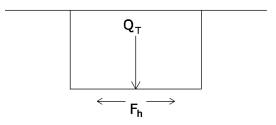When the vertical load is positive (push) the total will always be positive and therefore will always be a horizontal frictional resistance. However, when the vertical load is negative (pull) the total will also have to be positive, because if it were not the foundation would move upwards, but the total value will be smaller, closer, sometimes to a value similar to zero.

QT = – QV + PF + PP >≈ 0

The friction coefficient cf between the foundation and the ground below could be affected by water. This is the reason why foundations usually have a minimum depth (usually ≥ 80 cm) so that rain or surface water (irrigation, watering…) does not affect soil under the footing. Not only because of friction but also because it would affect the bearing capacity of the soil. If it is still possible that the ground under the footing can be wet (for example, groundwater) the coefficient cf may be close to zero.

Conversely, in most cases, concrete and soil interface is less than perfect, due to the process of pouring the concrete, so the friction coefficient can reach its maximum value cf = tan (Φ).

In short, the value of horizontal friction at the base of the foundation is:

Fh = cf · QT

2. Friction on the sides of the foundation

The sides of a foundation are subject to the earth pressure at rest. This value, as discussed above, is:

E0T = a · ½ · K0 · γ . h²

where a is the width of the face and h its height. In this case, too, there are several reasons for which this value could be zero.

• The vertical plane between the ground and the foundation is wet: by rainwater that has percolated, by water table, irrigation, pouring, etc.
• Contact between the earth and foundation does not exist because the soil has been separated from the foundation:
• due to the cohesion or the retraction of the soil (expansive clays, for example).
• due to excessive shrinkage of the concrete of the footing.
• due to the movement, albeit minimal, of the foundation because of the horizontal force (this case only affects the back of the footing, in the direction of motion).
• In the case of prefabricated foundations, with very smooth faces.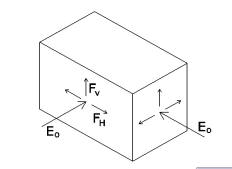In all these cases, if we can not determine the degree of contact and friction between concrete and soil, it’s better not to take into account this value to balance the external loads action. It is safer.

This type of lateral friction can take two directions:

• Vertical: The four faces of the footing. This is to avoid an upward motion. It’s taken into account to balance pulling vertical loads. It is not considered in a pushing load (downward vertical movement), as the ground below the footing always prevents this movement.
• Horizontal: it affects both sides of the foundation (assuming a rectangular footing). It is used to balance horizontal loads.

In short, if we consider a prismatic footing with dimensions (a x b) x h the total amount of horizontal friction at the sides of the footing, in a motion parallel to the face a or face b will be:

Fha = cf · a · K0 · γ . h²
Fhb = cf · b · K0 · γ . h²

In case of vertical motion (pulling loads), the maximum friction value will be

Fv = cf · (a+b) · K0 · γ . h²

But we must recall that in some cases lateral friction can be zero, due to some previous horizontal movements.

##### Self-weight

This is the most reliable resource for balance. The weight of the foundation is a value that does not change and that is always present. The weight of the constructive elements located above the footing can also be reliable (pavement, floors, concrete slabs, etc.). Although in some cases we must consider the possibility that these elements can be altered or withdrawn after a certain time, for any reason.

Most foundations are made with reinforced concrete. Depending on the quality of the concrete and the quality of the reinforcement, the relative density of the footing varies between 2.2 to 2.5. The same can be said of the possible concrete slab or reinforced concrete slab that may be found above the footing. Layers of gravel or paving have more variable densities but always with values between 1.5 and 2.

Given the reliability of this balance resource, it is advisable in some cases (e.g. pulling forces) to rely only on it to balance the load and let the other resources to achieve the safety factor. Anyway, this is not normative, although it is quite in line with common sense.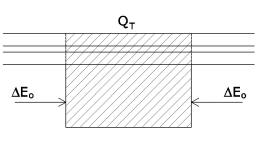The existence of continuous layers of earth, pavements, concrete slabs or floors on top of the the foundation is one more factor to take into account when assessing the weight of the foundation. Obviously, all these layers have a weight that is above the foundation and it must be added to the weight of this foundation, for all purposes.

Not only that: the existence of a uniform weight on the ground where the foundation is located increases the earth pressure at rest that we have discussed in previous sections. This increased value is a favourable factor, since it increases the bottom and lateral friction and the lateral resistance of the foundation in front of horizontal loads. However, nothing happens if we do not consider this increase in earth pressure at rest, since that puts us on the side of safety.

Nevertheless, in those cases when we need to refine the analysis, it is obvious that we can take into account this increase.

##### Reinforced concrete slab

In the design and analysis of foundations of tensile constructions, the existence of a structural concrete slab has a great importance. If it is not properly reinforced (following the current legislation) it can only be considered as part of the pavement. In this case it will contribute only with its own weight, with the area corresponding to the foundation.

But when we are sure of the existence of this reinforced concrete, all the time, it can contribute in a decisive manner in the dimensioning of the foundation. So much that it will require being very careful and demanding if we take it into consideration.

Basically, a slab of reinforced concrete can contribute to:

• Balancing the horizontal loads applied to the foundation. In many cases, if the horizontal component Fx of the extraction load is not large, and the pavement has a sufficiently reinforced concrete slab, the horizontal load is balanced by the horizontal resistance to compression RH of the slab. This compressive force must be compensated by the friction between the slab and the ground. That is, the slab must have dimensions large enough to balance the horizontal force.It can also be balanced by another opposite horizontal force applied to another foundation, which is quite usual in tensile constructions.Another point that requires consideration is the thickness of the slab. If this thickness is small, it could easily provoke a deformation of the slab by buckling. Fortunately, most paving slabs are thick enough to prevent the buckling, but it is a factor that can not be neglected at all.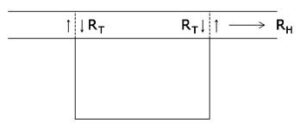• Balancing vertical pulling loads, through shear reaction RT in the section of the slab around the perimeter of the foundation.

Note:
We could go further and consider the perimeter P increased by half the slab depth on each side:

P = 2 · ((a+d)+(b+d))

If we don’t do it, we’re on the side of safety.

The shear resistance depends on the concrete and the reinforcement, but to ease the analysis, at the safety side, we can neglect the collaboration of the reinforcement. Therefore it will be only necessary to know the concrete shear strength τ and the effective depth d of the concrete slab at the perimeter of the foundation.

According to the local building standards we’ll determine the shear strength of concrete τ. The shear resistance of a concrete slab of thickness d is:

Rt = 2 · (a + b) · d · τ

It is possible that the value of the shear resistance of the slab might be much larger than the one produced by the dead weight (foundation, slab itself and the pavement) and the frictional resistance to extraction. That’s why we need to be very careful when using this value and be sure that:

• The total weight of the slab and pavement outside the shear zone is greater or equal to the shear force. Otherwise the pavement would rise before generating the resistance that has been calculated.
• When mobilizing this resistance, there is no bending moment on the slab causing not only breakage (which would nullify the shear resistance) but cracking the slab. If so, effective depth d would be severely diminished.

For example if we assume a case where a = b = 1m; d = 0.10 m; τ = 5 kg/cm², value of Rt would be 20 t. To generate a shear resistance of 20 tonnes it would be necessary to mobilize an about 45 m2 (6.8 x6.8) slab surface. This would make the centre of gravity of the trapezoid corresponding to each face pavement to be more or less at about 2 m from the perimeter of the foundation.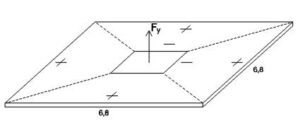As in every face a load of 20 / 4 = 5 t is applied, the moment that should support the concrete section (b x h = 100 x 10 cm) would be M = 5 x 2 = 10 mt.
If this value is unacceptable for the concrete section or by the existing reinforcement, as it seems obvious, it will be necessary to reconsider the shear strength of 20 t provided by the slab, and to check, iteratively, which is the maximum value that can be assumed by the existing reinforced concrete slab.

A sensible recommendation would be not to use more than half the thickness d of the slab for an element that generates resistance to shear forces. In this way we give quite a large margin of possible cracking. But the best solution is to study the flexural behaviour of the slab and see what the real thickness is of the concrete uncracked section at the perimeter of the foundation.

##### The reinforced concrete slab as single foundation

A slab of reinforced concrete can be the only foundation in some extraordinary cases. These cases must be careful considered. This slab can support typical tensile construction loads: horizontal forces, vertical forces (pulling and pushing) and bending moments.

It is a normal situation to have to build on an existing reinforced concrete slab, and the question arises about whether this slab by itself is or is not able to behave as the foundation. Some other times this slab does not exist, but we need to do it for project issues.

The biggest difference between a slab foundation and footings or piles is the own deformation. For a slab, this deformation can be:

• upwards, off the floor, so that horizontal friction forces decrease, being necessary to check that the shear anchorage resistance of the slab is enough.
• downwards, generating a different ground reaction at each point of the slab (theory of elastic supports) and also checking the punching of the slab.

In any of these two cases and in general in all reinforced concrete slab foundations, the reinforcement of the slab plays an important role. Normally the slab is not too thick, and the reinforcement protection must be large (the soil at one side and the open sky at the other), so the mechanical effective depth is quite small. This makes it difficult to determine the deformations of the slab, since cracking will produce many changes in the real stiffness of the slab.

El terreno, Matilde González Caballero, Edicions UPC, 2001